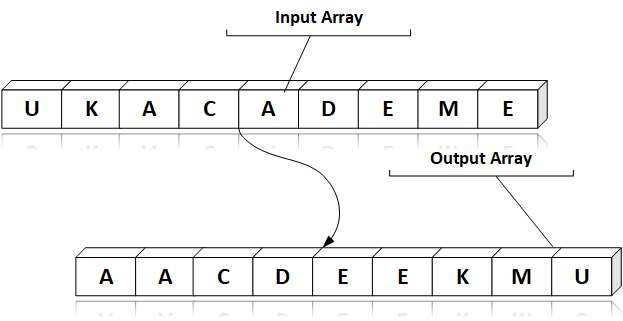# Array C Programing

Answer: Sorting is the process of arranging the elements of the array in some logical order. This logical order may be ascending or descending in case of numeric values or dictionary order in case of alphanumeric values. Bubble sort is a technique to sort the elements of array.

Example: Increasing order of ASCII values is sorted from the following character list. That is, the lower ASCII character is put first than the greater ASCII-value character.Sorting can be done with a number of techniques:

• Bubble Sort
• Insertion Sort
• Selection Sort
• Quick Sort
• Merge Sort
• Heap Sort

Bubble Sort

Bubble Sort is a simple algorithm used to sort a set of n elements, supplied in the form of an array of n elements. Bubble Sort compares the whole element one by one and sortes it according to its values.

Insertion Sort

Sorting is done through the insertion of items in an existing sorted list. At first, there was only one item in the sorted list. In the proper position, other elements will be progressively added to the list.

Selection Sort

This algorithm will first discover the lowest component in the set and replace it with the element in the first place, and then locate the second lowest element, replace it with the element in the second place.

Quick Sort

Quick sort is a very effective sorting algorithm based on the distribution of data array into smaller arrays. A big range is partitioned into two sections. One array contains values that are less than the given value (i.e. pivot) and another array contains values that are larger than the pivot value.

Merge Sort

Merge is a dividing and conquering technique based sorting technique. With O(n log n), the worst-case complexity is one of the most respected algorithms.

Heap Sort

Heap sort type is a comparative sorting technique based upon the structure of the binary heap data. It's similar to a sort where the maximum element is to be found first and the maximum element is placed at the end. The same process is repeated for the rest of the element.